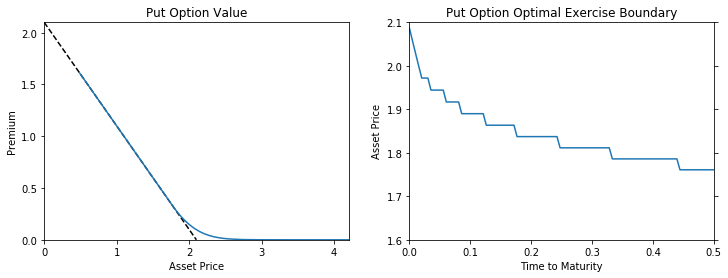# DiscreteDP Example: Option Pricing¶

Daisuke Oyama

Faculty of Economics, University of Tokyo

From Miranda and Fackler, Applied Computational Economics and Finance, 2002, Section 7.6.4

In :
%matplotlib inline

In :
import numpy as np
from scipy import sparse
import matplotlib.pyplot as plt
import quantecon as qe
from quantecon.markov import DiscreteDP, backward_induction, sa_indices

In :
T = 0.5       # Time expiration (years)
vol = 0.2     # Annual volatility
r = 0.05      # Annual interest rate
strike = 2.1  # Strike price
p0 = 2        # Current price
N = 100       # Number of periods to expiration

# Time length of a period
tau = T/N

# Discount factor
beta = np.exp(-r*tau)

# Up-jump factor
u = np.exp(vol*np.sqrt(tau))

# Up-jump probability
q = 1/2 + np.sqrt(tau)*(r - (vol**2)/2)/(2*vol)


We follow the state-action pairs formulation approach. We let the state space consist of the possible values of the asset price and the state indicating that "the option has been exercised".

In :
# Possible price values
ps = u**np.arange(-N, N+1) * p0

# Number of states
n = len(ps) + 1  # State n-1: "the option has been exercised"

# Number of actions
m = 2  # 0: hold, 1: exercise

# Number of feasible state-action pairs
L = n*m - 1  # At state n-1, there is only one action "do nothing"

In :
# Arrays of state and action indices
s_indices, a_indices = sa_indices(n, m)
s_indices, a_indices = s_indices[:-1], a_indices[:-1]

In :
# Reward vector
R = np.empty((n, m))
R[:, 0] = 0
R[:-1, 1] = strike - ps
R = R.ravel()[:-1]

In :
# Transition probability array
Q = sparse.lil_matrix((L, n))
for i in range(L-1):
if a_indices[i] == 0:
Q[i, min(s_indices[i]+1, len(ps)-1)] = q
Q[i, max(s_indices[i]-1, 0)] = 1 - q
else:
Q[i, n-1] = 1
Q[L-1, n-1] = 1

In :
# Create a DiscreteDP
ddp = DiscreteDP(R, Q, beta, s_indices, a_indices)


The backward induction algorithm for finite horizon dynamic programs is offered as the function backward_induction. (By default, the terminal value function is set to the vector of zeros.)

In :
vs, sigmas = backward_induction(ddp, N)


In the returns, vs is an $(N+1) \times n$ array that contains the optimal value functions, where vs is the value vector at the current period (i.e., period $0$) for different prices (with the value vs[0, -1] = 0 for the state "the option has been exercised" included), and sigmas is an $N \times n$ array that contins the optimal policy functions.

In :
v = vs
max_exercise_price = ps[sigmas[::-1].sum(-1)-1]

In :
fig, axes = plt.subplots(1, 2, figsize=(12, 4))

axes.plot([0, strike], [strike, 0], 'k--')
axes.plot(ps, v[:-1])
axes.set_xlim(0, strike*2)
axes.set_xticks(np.linspace(0, 4, 5, endpoint=True))
axes.set_ylim(0, strike)
axes.set_yticks(np.linspace(0, 2, 5, endpoint=True))
axes.set_xlabel('Asset Price')
axes.set_title('Put Option Value')

axes.plot(np.linspace(0, T, N), max_exercise_price)
axes.set_xlim(0, T)
axes.set_ylim(1.6, strike)
axes.set_xlabel('Time to Maturity')
axes.set_ylabel('Asset Price')
axes.set_title('Put Option Optimal Exercise Boundary')
axes.tick_params(right='on')

plt.show()The figure in the right panel looks different from the corresponding figure in Miranda and Fackler (Figure 7.4(b), p.180).

In [ ]: